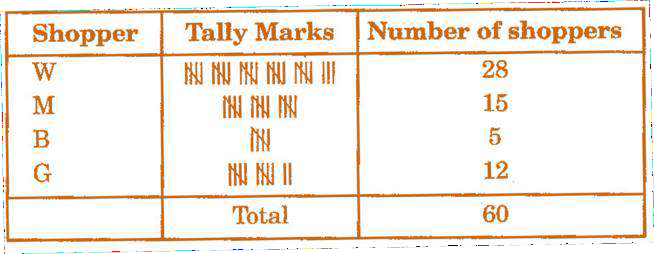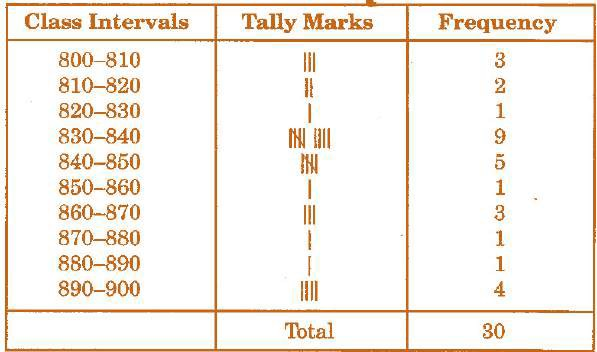# Data Handling Chapter 5 Class 8 Maths NCERT Textbook With Solutions PDF

NCERT Solutions for Class 8 Maths Chapter 5′ PDF Quick download link is given at the bottom of this article. You can see the PDF demo, size of the PDF, page numbers, and direct download Free PDF of ‘Ncert Class 8 Maths Chapter 5 Exercise Solution’ using the download button.

### Chapter 5:Data Handling

#### 5.1 Looking for Information

In your day-to-day life, you might have come across information, such as:
(a) Runs made by a batsman in the last 10 test matches.
(b) Number of wickets taken by a bowler in the last 10 ODIs.
(c) Marks scored by the students of your class in the Mathematics unit test.

To find this, she will write the heights of all the students in her class, organize the data in a systematic manner and then interpret it accordingly.

Sometimes, data is represented graphically to give a clear idea of what it represents. Do you remember the different types of graphs which we have learned in earlier classes?

#### 5.2 Organising Data

Usually, data available to us is in an unorganized form called raw data. To draw meaningful inferences, we need to organize the data systematically.

For example, a group of students was asked about their favorite subject.

The results were as listed below: Art, Mathematics, Science, English, Mathematics, Art, English, Mathematics, English, Art, Science, Art, Science, Science, Mathematics, Art, English, Art, Science, Mathematics, Science, Art.

Which is the most liked subject and the one least liked? 72 MATHEMATICS TRY THESE
It is not easy to answer the question by looking at the choices written haphazardly. We arrange the data in Table 5.1 using tally marks.

### NCERT Solutions Class 8 Maths Chapter 5 Data Handling

1. For which of these would you use a histogram to show the data?

(a) The number of letters for different areas in a postman’s bag.

(b) The height of competitors in an athletics meet.

(c) The number of cassettes produced by 5 companies.

(d) The number of passengers boarding trains from 7.00 a.m. to 7.00 p.m. at a station. Give reason for each.

Solution:

We know that a Histogram is a graphical representation of data if the data is represented using class- interval.

Since the cases mentioned in options (b) and (d) can be divided into class intervals, the histogram can be used to show the data.

Similarly, since the cases mentioned in options (a) and (c) cannot be divided into class intervals, the histogram cannot be used to represent the data.

2. The shoppers who come to a departmental store are marked as: man (M), woman (W), boy (B), or girl (G). The following list gives the shoppers who came during the first hour in the morning.

W W W G B W W M G G M M W W W W G B M W B G G M W W M M W W W M W B W G M W W W W G W M M W M W G W M G W M M B G G W.

Make a frequency distribution table using tally marks. Draw a bar graph to illustrate it.

Solution:Frequency distribution table:

Bar-graph:

3. The weekly wages (in ₹) of 30 workers in a factory are:

830, 835, 890, 810, 835, 836, 869, 845, 898, 890, 820, 860, 832, 833, 855, 845, 804, 808,

812, 840, 885, 835, 835, 836, 878, 840, 868, 890, 806, 840.

Using tally marks, make a frequency table with intervals as 800 – 810, 810 – 820, and so on.

Solution:The frequency table with intervals as 800 – 810, 810 – 820 and so on, using tally marks is given below:

4. Draw a histogram for the frequency table made for the data in Question 3 and answer the following questions.

(i) Which group has the maximum number of workers?

(ii) How many workers earn ₹ 850 and more?

(iii) How many workers earn less than ₹ 850?

Solution:

(i) 830-840 is the group having the maximum number of workers, 9, compared to other groups.

(ii) Workers earning ₹ 850 and more= 1+3+1+1+4=10

(iii) Workers earning less than ₹ 850= 3+2+1+9+5=20

5. The number of hours for which students of a particular class watched television during holidays is shown through the given graph.

(i) For how many hours did the maximum number of students watch T.V.?

(ii) How many students watched TV for less than 4 hours?

(iii) How many students spent more than 5 hours in watching TV?

Solution:

(i) 32 students watched T.V for 4-5 hours. ∴, The maximum number of students who watched T.V. for 4 – 5 hours.

(ii) The number of students who watched T.V. less than 4 hours= 22+8+4=34

(iii) The number of students who spent more than 5 hours in watching TV

=8+6=14